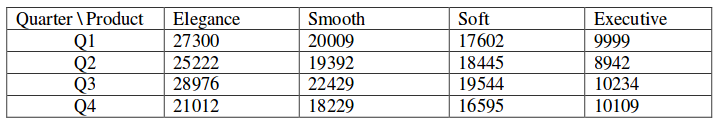GFG App
Open AppBrowser
Continue

# GATE | GATE-CS-2016 (Set 1) | Question 6

A shaving set company sells 4 different types of razors, Elegance, Smooth, Soft and Executive. Elegance sells at Rs. 48, Smooth at Rs. 63, Soft at Rs. 78 and Executive at Rs. 173 per piece. The table below shows the numbers of each razor sold in each quarter of a year.Which product contributes the greatest fraction to the revenue of the company in that year?

(A) Elegance
(B) Executive
(C) Smooth
(D) Soft

Explanation: Revenue from Elegance = (27300+25222+28976+21012) * Rs. 48 = Rs. 4920480
Revenue from Smooth = (20009+19392+22429+18229) * Rs. 63 = Rs. 5043717
Revenue from Soft = (17602+18445+19544+16595) * Rs. 78 = Rs. 5630508
Revenue from Executive = (9999+8942+10234+10109) * Rs. 173 = Rs. 6796132
Total Revenue = Rs. 22390837

Fraction of Revenue for Elegance = 0.219
Fraction of Revenue for Smooth = 0.225
Fraction of Revenue for Soft = 0.251
Fraction of Revenue for Executive = 0.303

Thus, B (Executive) is the correct answer.

Quiz of this Question

My Personal Notes arrow_drop_up